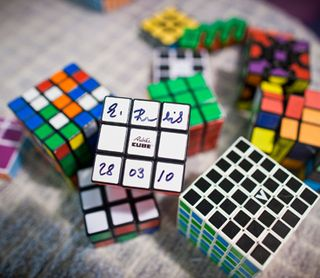# Math Uncovers Max Moves to Solve Big Rubik's CubesThe brain twister that is the Rubik's cube has not only befuddled many people that have tried to solve it, but it has also stumped mathematicians.

Earlier this year, researchers deciphered the classic Rubik's cube, which has nine squares per side (three per edge of the cube) and six different colors, calculating that from any of the 43 quintillion possible orientations, the cube could be solved in fewer than 20 moves. A "solved" Rubik's cube has only one color of squares on each of its six faces.

Figuring this out took the equivalent of 35 years' worth of number crunching on a home desktop computer. Researchers at MIT, led by Erik Demaine, needed to figure out all of the cube's possible starting positions before they could understand each of the solutions. Doing the same for other similar math puzzles, say one with four or five squares per edge, would take more computing time than all the world's computers.

Instead of approaching the problem from the starting point, the team figured out how the number of squares per edge of the cube changes the maximum number of moves needed to solve it. [Twisted Physics: 7 Mind-Blowing Findings]Team led by Erik Demaine figuring out the mathmatics of the Rubik's cube. From left to right, Sarah Eisenstat, Martin Demaine, Erik Demaine and Andrew Winslow. (Image credit: Dominick Reuter)

What they found was surprising. Instead of the result they expected, that the maximum moves needed to solve a cube with X squares per side is proportional to X-squared, the answer they got was that it was proportional to X-squared divided by the logarithm of X, or X2/logX, a number larger than just squaring X.

Why the difference? Traditionally, the puzzles are solved by moving one square into position at a time, while leaving the rest of the squares in place. In reality, each twist has the potential to move multiple squares into position, not just one.

It took months for the team to prove that the "X2/logX" equation equals the maximum number of moves from every possible starting configuration. Their calculations are still a little off, though, as their computer simulation always overestimates the number of moves required.

The proofs and calculations Demaine and his team developed to figure out the puzzle of the Rubik's cube could also be used for other cool math games and configuration-based problems, such as having to reorganize boxes in a warehouse.

"My life has been driven by solving problems that I consider fun," Demaine said in a statement. "It’s always hard to tell at the moment what is going to be important. Studying prime numbers was just a recreational activity. There was no practical importance to that for hundreds of years until cryptography came along."

A short version of this paper is set to appear at the 19th Annual European Symposium on Algorithms, which takes place in September.